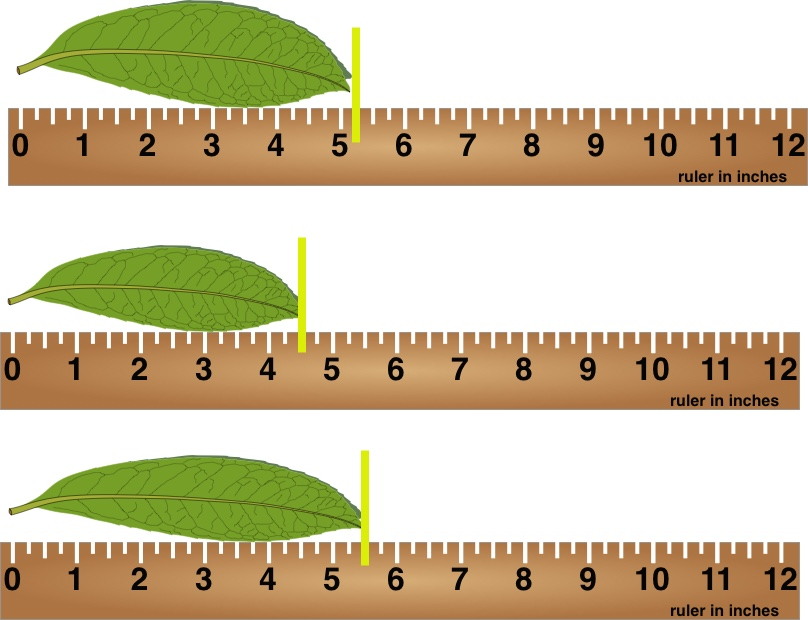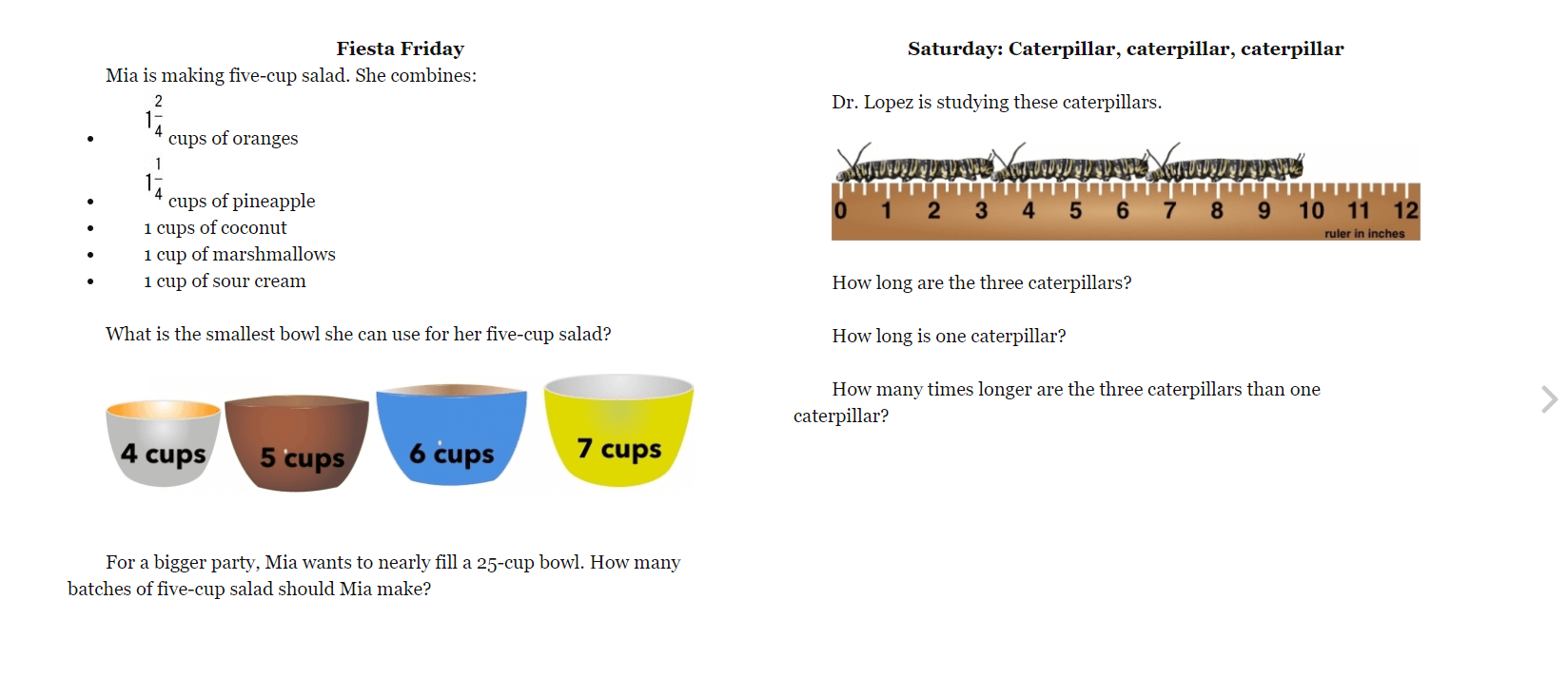# Summer Mathness 5

Welcome to the Summer Mathness 5 page!

You can always buy the book at Amazon.You can enter comments below that link to pictures, YouTube videos and descriptions of your answer and how you found it. Please also support and give good feedback to others working on these problems.

Please note that we will not post our feedback and solutions before the day listed in your book.

Thanks and happy summer!

## 8 thoughts on “Summer Mathness 5”

1. Thursday. 6/16

4 quarters is \$1. So 12 quarters is \$3.

1. the weekend warrior walks 5×7=35

and, 4.5×7=28+3.5 which is 31.5

So, the difference is 3.5 miles (3.5+31.5=35.0).

2. the 2 tomatoes are 4 quarters which is \$1. The peppers and onions are 8 quarters, which is \$2.

The total is \$3.

2. Wed. 6/15

I subtracted (2.5+2.5)-4.5 = 0.5

So after 7 weeks, the difference is 7×0.5=3.5 miles.

1. the weekend warrior walks 5×7=35

and, 4.5×7=28+3.5 which is 31.5

So, the difference is 3.5 miles (3.5+31.5=35.0).

3. June 14 Tuesday

55 feet
X. 55 feet
3025 square feet

1. I did this in my head because 55×5=275. So, 55×50=2750. So the total area is 2750+275=3025 square feet

4. June 13, Monday

I Divided and got 4 and 5.5